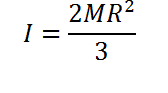# Moment of Inertia of a Hollow Sphere Calculator

This CalcTown Calculator calculates the Moment of Inertia of a Hollow Sphere along an axis passing through its centre.

kg
m

#### Result

Kg-m2where

M is the mass of the Sphere

R is the radius of the sphere.